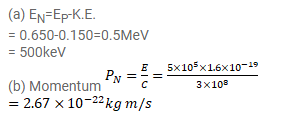# Prove the following

Question:

In the decay ${ }^{64} \mathrm{Cu} \rightarrow{ }^{64} \mathrm{Ni}+\mathrm{e}^{+}+\mathrm{v}$, the maximum kinetic energy carried by the position is found to be $0.650 \mathrm{MeV}$.

(a) What is the energy of the neutrino which was emitted together with a positron of kinetic energy $0.150 \mathrm{MeV}$ ?

(b) What is the momentum of this neutrino in $\mathrm{kg}-\mathrm{m} / 3$ ? Use the formula applicable to a photon.

Solution: